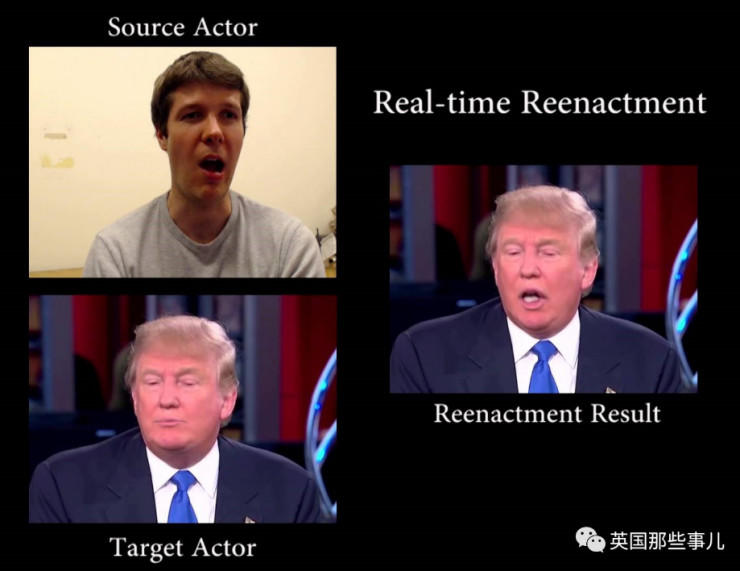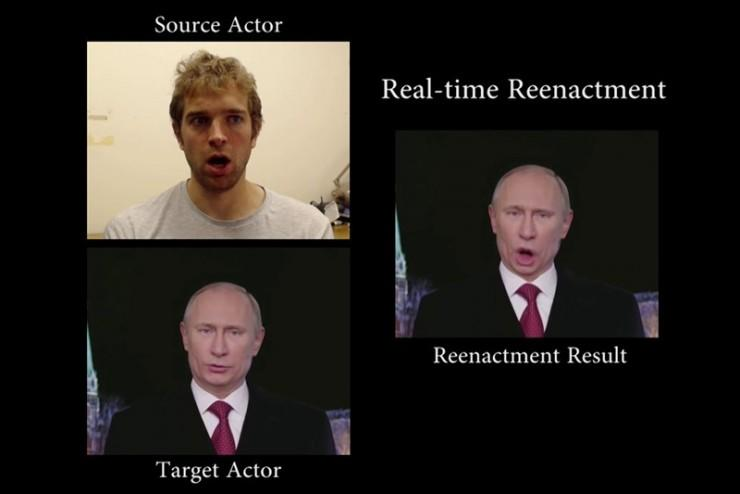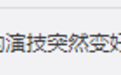# AI 让朱茵秒变杨幂，但我拒绝成为波多野结衣

2019年02月28日 15:58:00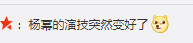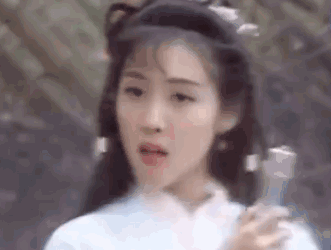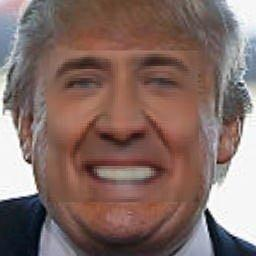1.收集凯奇的照片并选择要修改的视频区域。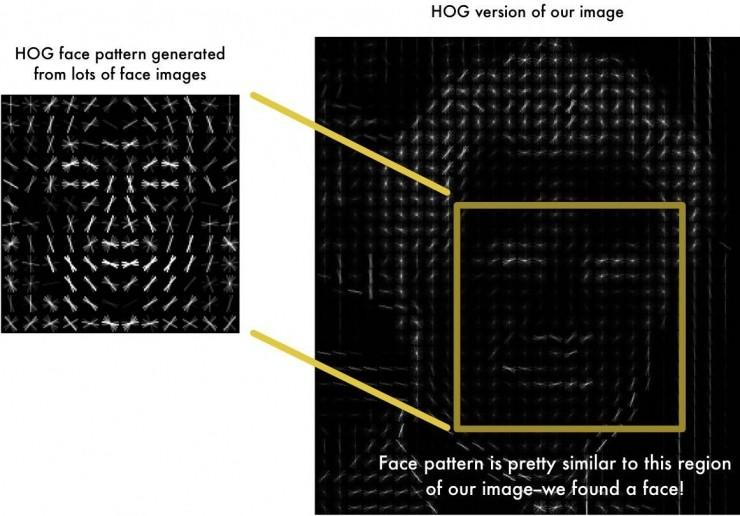2.使用方向梯度直方图（简称HOG）得到凯奇的脸部特征。

3.使用特征数据，训练一个自动编码器。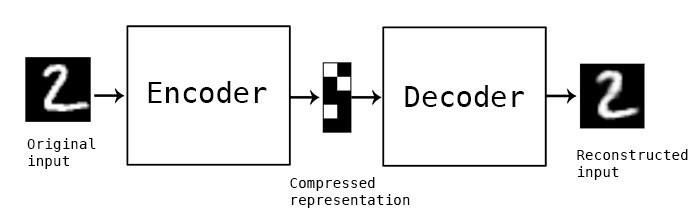Deepfake 的整个流程包括三步，一是提取数据，二是训练，三是转换。其中第一和第三步都需要用到数据预处理，另外第三步还用到了图片融合技术。所以我在技术上主要分三个方面来剖析：图像预处理、网络模型、图像融合。

1. 图像预处理

2. 网络模型

Encoder: 64x64x3->8x8x512
x = input_
x = conv(128)(x)
x = conv(256)(x)
x = conv(512)(x)
x = conv(1024)(x)
x = Dense(ENCODER_DIM)(Flatten()(x))
x = Dense(4 * 4 * 1024)(x)
x = Reshape((4, 4, 1024))(x)
x = upscale(512)(x)

Decoder：8x8x512->64x64x3
x = input_
x = upscale(256)(x)
x = upscale(128)(x)
x = upscale(64)(x)
x = Conv2D(3, kernel_size=5, padding='same', activation='sigmoid')(x)

def conv(filters):
def block(x):
x = Conv2D(filters, kernel_size=5, strides=2, padding='same')(x)
x = LeakyReLU(0.1)(x)
return x
return blockdef upscale(filters):
def block(x):
x = Conv2D(filters * 4, kernel_size=3, padding='same')(x)
x = LeakyReLU(0.1)(x)
x = PixelShuffler()(x)
return x
return block

conv 是中规中矩的卷积加 relu 激活函数，upscale 中有个函数叫 PixelShuffler，这个函数很有意思，其功能是将 filter 的大小变为原来的 1/4，让后让高 h、宽 w 各变为原来的两倍，这也就是为什么前面的卷积层的 filter 要乘以 4 的原因。

3. 图像融合